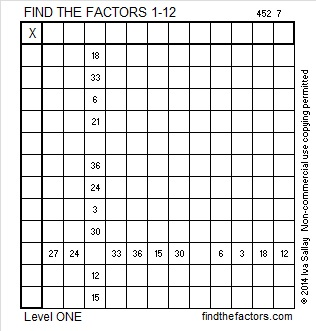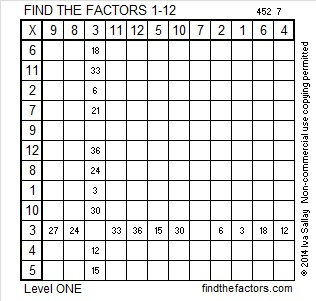# 335 and Level 1

• 335 is a composite number.
• Prime factorization: 335 = 5 x 67
• The exponents in the prime factorization are 1 and 1. Adding one to each and multiplying we get (1 + 1)(1 + 1) = 2 x 2 = 4. Therefore 335 has exactly 4 factors.
• Factors of 335: 1, 5, 67, 335
• Factor pairs: 335 = 1 x 335 or 5 x 67
• 335 has no square factors that allow its square root to be simplified. √335 ≈ 18.303If you can multiply, divide and use a little logic, you should easily be able to complete this multiplication table puzzle.Print the puzzles or type the factors on this excel file: 12 Factors 2014-12-29